Search by Topic

Resources tagged with Visualising similar to ABC:

Filter by: Content type:
Age range:
Challenge level:

There are 256 results

Broad Topics > Using, Applying and Reasoning about Mathematics > VisualisingWaiting for Blast Off

Age 7 to 11 Challenge Level:

10 space travellers are waiting to board their spaceships. There are two rows of seats in the waiting room. Using the rules, where are they all sitting? Can you find all the possible ways?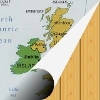Map Folding

Age 7 to 11 Challenge Level:

Take a rectangle of paper and fold it in half, and half again, to make four smaller rectangles. How many different ways can you fold it up?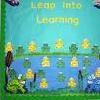Display Boards

Age 7 to 11 Challenge Level:

Design an arrangement of display boards in the school hall which fits the requirements of different people.Coded Hundred Square

Age 7 to 11 Challenge Level:

This 100 square jigsaw is written in code. It starts with 1 and ends with 100. Can you build it up?Neighbours

Age 7 to 11 Challenge Level:

In a square in which the houses are evenly spaced, numbers 3 and 10 are opposite each other. What is the smallest and what is the largest possible number of houses in the square?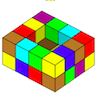Painting Possibilities

Age 7 to 11 Challenge Level:

This task, written for the National Young Mathematicians' Award 2016, involves open-topped boxes made with interlocking cubes. Explore the number of units of paint that are needed to cover the boxes. . . .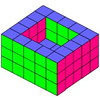Open Boxes

Age 7 to 11 Challenge Level:

Can you work out how many cubes were used to make this open box? What size of open box could you make if you had 112 cubes?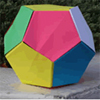Dodecamagic

Age 7 to 11 Challenge Level:

Here you see the front and back views of a dodecahedron. Each vertex has been numbered so that the numbers around each pentagonal face add up to 65. Can you find all the missing numbers?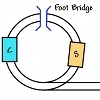Shunting Puzzle

Age 7 to 11 Challenge Level:

Can you shunt the trucks so that the Cattle truck and the Sheep truck change places and the Engine is back on the main line?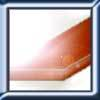Little Boxes

Age 7 to 11 Challenge Level:

How many different cuboids can you make when you use four CDs or DVDs? How about using five, then six?Single Track

Age 7 to 11 Challenge Level:

What is the best way to shunt these carriages so that each train can continue its journey?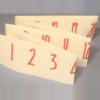Folded Number Line

Age 7 to 11 Challenge Level:

When I fold a 0-20 number line, I end up with 'stacks' of numbers on top of each other. These challenges involve varying the length of the number line and investigating the 'stack totals'.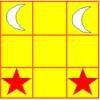Knight's Swap

Age 7 to 11 Challenge Level:

Swap the stars with the moons, using only knights' moves (as on a chess board). What is the smallest number of moves possible?Putting Two and Two Together

Age 7 to 11 Challenge Level:

In how many ways can you fit two of these yellow triangles together? Can you predict the number of ways two blue triangles can be fitted together?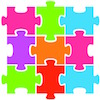Jigsaw Pieces

Age 7 to 11 Challenge Level:

How will you go about finding all the jigsaw pieces that have one peg and one hole?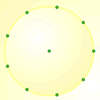Age 7 to 11 Challenge Level:

How many DIFFERENT quadrilaterals can be made by joining the dots on the 8-point circle?Domino Numbers

Age 7 to 11 Challenge Level:

Can you see why 2 by 2 could be 5? Can you predict what 2 by 10 will be?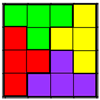Tetrafit

Age 7 to 11 Challenge Level:

A tetromino is made up of four squares joined edge to edge. Can this tetromino, together with 15 copies of itself, be used to cover an eight by eight chessboard?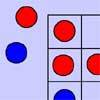Red Even

Age 7 to 11 Challenge Level:

You have 4 red and 5 blue counters. How many ways can they be placed on a 3 by 3 grid so that all the rows columns and diagonals have an even number of red counters?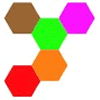Hexpentas

Age 5 to 11 Challenge Level:

How many different ways can you find of fitting five hexagons together? How will you know you have found all the ways?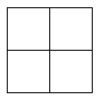Two Squared

Age 7 to 11 Challenge Level:

What happens to the area of a square if you double the length of the sides? Try the same thing with rectangles, diamonds and other shapes. How do the four smaller ones fit into the larger one?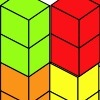Triple Cubes

Age 5 to 11 Challenge Level:

This challenge involves eight three-cube models made from interlocking cubes. Investigate different ways of putting the models together then compare your constructions.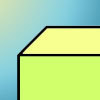Cuboid-in-a-box

Age 7 to 11 Challenge Level:

What is the smallest cuboid that you can put in this box so that you cannot fit another that's the same into it?Four Triangles Puzzle

Age 5 to 11 Challenge Level:

Cut four triangles from a square as shown in the picture. How many different shapes can you make by fitting the four triangles back together?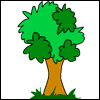Paw Prints

Age 7 to 11 Challenge Level:

A dog is looking for a good place to bury his bone. Can you work out where he started and ended in each case? What possible routes could he have taken?Nine-pin Triangles

Age 7 to 11 Challenge Level:

How many different triangles can you make on a circular pegboard that has nine pegs?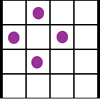Square Corners

Age 7 to 11 Challenge Level:

What is the greatest number of counters you can place on the grid below without four of them lying at the corners of a square?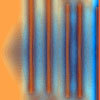Move a Match

Age 7 to 11 Challenge Level:

How can you arrange these 10 matches in four piles so that when you move one match from three of the piles into the fourth, you end up with the same arrangement?Clocked

Age 11 to 14 Challenge Level:

Is it possible to rearrange the numbers 1,2......12 around a clock face in such a way that every two numbers in adjacent positions differ by any of 3, 4 or 5 hours?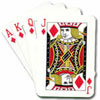Counting Cards

Age 7 to 11 Challenge Level:

A magician took a suit of thirteen cards and held them in his hand face down. Every card he revealed had the same value as the one he had just finished spelling. How did this work?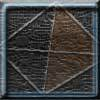Tetrahedron Faces

Age 7 to 11 Challenge Level:

One face of a regular tetrahedron is painted blue and each of the remaining faces are painted using one of the colours red, green or yellow. How many different possibilities are there?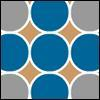Counters

Age 7 to 11 Challenge Level:

Hover your mouse over the counters to see which ones will be removed. Click to remove them. The winner is the last one to remove a counter. How you can make sure you win?Christmas Chocolates

Age 11 to 14 Challenge Level:

How could Penny, Tom and Matthew work out how many chocolates there are in different sized boxes?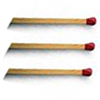Matchsticks

Age 7 to 11 Challenge Level:

Reasoning about the number of matches needed to build squares that share their sides.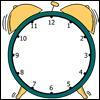A Square in a Circle

Age 7 to 11 Challenge Level:

What shape has Harry drawn on this clock face? Can you find its area? What is the largest number of square tiles that could cover this area?Odd Squares

Age 7 to 11 Challenge Level:

Think of a number, square it and subtract your starting number. Is the number you’re left with odd or even? How do the images help to explain this?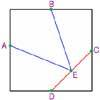Midpoint Triangle

Age 7 to 11 Challenge Level:

Can you cut up a square in the way shown and make the pieces into a triangle?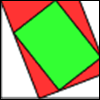Folding, Cutting and Punching

Age 7 to 11 Challenge Level:

Exploring and predicting folding, cutting and punching holes and making spirals.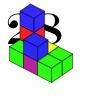28 and It's Upward and Onward

Age 7 to 11 Challenge Level:

Can you find ways of joining cubes together so that 28 faces are visible?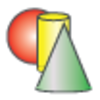Sponge Sections

Age 7 to 11 Challenge Level:

You have been given three shapes made out of sponge: a sphere, a cylinder and a cone. Your challenge is to find out how to cut them to make different shapes for printing.Making Maths: Rolypoly

Age 5 to 11 Challenge Level:

Paint a stripe on a cardboard roll. Can you predict what will happen when it is rolled across a sheet of paper?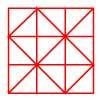Fractional Triangles

Age 7 to 11 Challenge Level:

Use the lines on this figure to show how the square can be divided into 2 halves, 3 thirds, 6 sixths and 9 ninths.Construct-o-straws

Age 7 to 11 Challenge Level:

Make a cube out of straws and have a go at this practical challenge.Tetrahedra Tester

Age 11 to 14 Challenge Level:

An irregular tetrahedron is composed of four different triangles. Can such a tetrahedron be constructed where the side lengths are 4, 5, 6, 7, 8 and 9 units of length?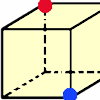Redblue

Age 7 to 11 Challenge Level:

Investigate the number of paths you can take from one vertex to another in these 3D shapes. Is it possible to take an odd number and an even number of paths to the same vertex?Multiplication Series: Illustrating Number Properties with Arrays

Age 5 to 11

This article for teachers describes how modelling number properties involving multiplication using an array of objects not only allows children to represent their thinking with concrete materials,. . . .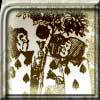Painting Cubes

Age 11 to 14 Challenge Level:

Imagine you have six different colours of paint. You paint a cube using a different colour for each of the six faces. How many different cubes can be painted using the same set of six colours?Cubes Within Cubes

Age 7 to 14 Challenge Level:

We start with one yellow cube and build around it to make a 3x3x3 cube with red cubes. Then we build around that red cube with blue cubes and so on. How many cubes of each colour have we used?Cubes Within Cubes Revisited

Age 11 to 14 Challenge Level:

Imagine starting with one yellow cube and covering it all over with a single layer of red cubes, and then covering that cube with a layer of blue cubes. How many red and blue cubes would you need?Colour Wheels

Age 7 to 11 Challenge Level:

Imagine a wheel with different markings painted on it at regular intervals. Can you predict the colour of the 18th mark? The 100th mark?